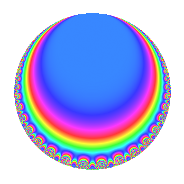Properties

 Label 128.3.d.aLevel 128 Weight 3 Character orbit 128.d Analytic conductor 3.488 Analytic rank 0 Dimension 2 CM disc. -4 Inner twists 4

Related objects

Newspace parameters

 Level: $$N$$ = $$128 = 2^{7}$$ Weight: $$k$$ = $$3$$ Character orbit: $$[\chi]$$ = 128.d (of order $$2$$ and degree $$1$$)

Newform invariants

 Self dual: No Analytic conductor: $$3.48774738381$$ Analytic rank: $$0$$ Dimension: $$2$$ Coefficient field: $$\Q(\sqrt{-1})$$ Coefficient ring: $$\Z[a_1, \ldots, a_{5}]$$ Coefficient ring index: $$2^{3}$$ Sato-Tate group: $\mathrm{U}(1)[D_{2}]$

$q$-expansion

Coefficients of the $$q$$-expansion are expressed in terms of $$i = \sqrt{-1}$$. We also show the integral $$q$$-expansion of the trace form.

 $$f(q)$$ $$=$$ $$q$$ $$+ \beta q^{5}$$ $$-9 q^{9}$$ $$+O(q^{10})$$ $$q$$ $$+ \beta q^{5}$$ $$-9 q^{9}$$ $$+ 3 \beta q^{13}$$ $$+ 30 q^{17}$$ $$-39 q^{25}$$ $$-5 \beta q^{29}$$ $$-3 \beta q^{37}$$ $$+ 18 q^{41}$$ $$-9 \beta q^{45}$$ $$+ 49 q^{49}$$ $$-7 \beta q^{53}$$ $$+ 15 \beta q^{61}$$ $$-192 q^{65}$$ $$+ 110 q^{73}$$ $$+ 81 q^{81}$$ $$+ 30 \beta q^{85}$$ $$+ 78 q^{89}$$ $$-130 q^{97}$$ $$+O(q^{100})$$ $$\operatorname{Tr}(f)(q)$$ $$=$$ $$2q$$ $$\mathstrut -\mathstrut 18q^{9}$$ $$\mathstrut +\mathstrut O(q^{10})$$ $$2q$$ $$\mathstrut -\mathstrut 18q^{9}$$ $$\mathstrut +\mathstrut 60q^{17}$$ $$\mathstrut -\mathstrut 78q^{25}$$ $$\mathstrut +\mathstrut 36q^{41}$$ $$\mathstrut +\mathstrut 98q^{49}$$ $$\mathstrut -\mathstrut 384q^{65}$$ $$\mathstrut +\mathstrut 220q^{73}$$ $$\mathstrut +\mathstrut 162q^{81}$$ $$\mathstrut +\mathstrut 156q^{89}$$ $$\mathstrut -\mathstrut 260q^{97}$$ $$\mathstrut +\mathstrut O(q^{100})$$

Character Values

We give the values of $$\chi$$ on generators for $$\left(\mathbb{Z}/128\mathbb{Z}\right)^\times$$.

 $$n$$ $$5$$ $$127$$ $$\chi(n)$$ $$-1$$ $$-1$$

Embeddings

For each embedding $$\iota_m$$ of the coefficient field, the values $$\iota_m(a_n)$$ are shown below.

For more information on an embedded modular form you can click on its label.

Label $$\iota_m(\nu)$$ $$a_{2}$$ $$a_{3}$$ $$a_{4}$$ $$a_{5}$$ $$a_{6}$$ $$a_{7}$$ $$a_{8}$$ $$a_{9}$$ $$a_{10}$$
63.1
 − 1.00000i 1.00000i
0 0 0 8.00000i 0 0 0 −9.00000 0
63.2 0 0 0 8.00000i 0 0 0 −9.00000 0
 $$n$$: e.g. 2-40 or 990-1000 Significant digits: Format: Complex embeddings Normalized embeddings Satake parameters Satake angles

Inner twists

Char. orbit Parity Mult. Self Twist Proved
1.a Even 1 trivial yes
4.b Odd 1 CM by $$\Q(\sqrt{-1})$$ yes
8.b Even 1 yes
8.d Odd 1 yes

Hecke kernels

This newform can be constructed as the kernel of the linear operator $$T_{3}$$ acting on $$S_{3}^{\mathrm{new}}(128, [\chi])$$.